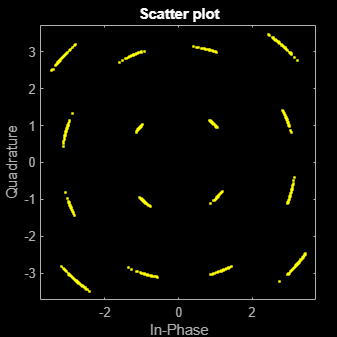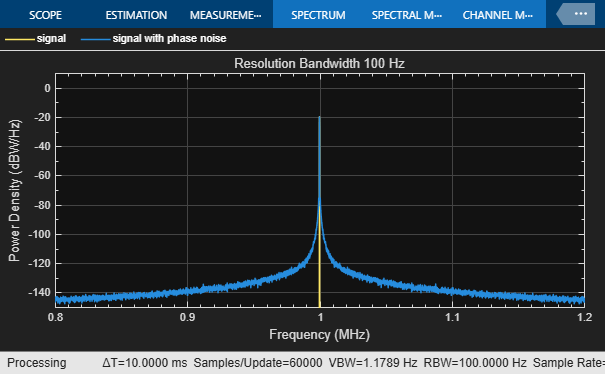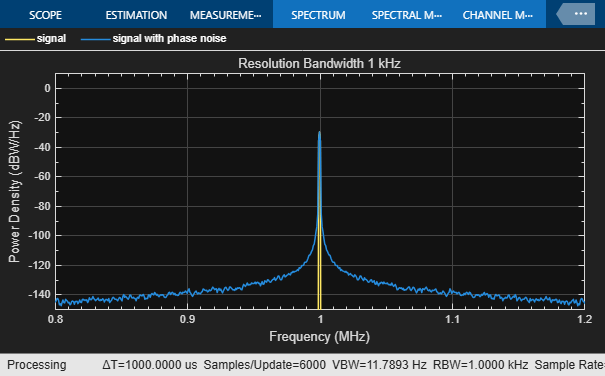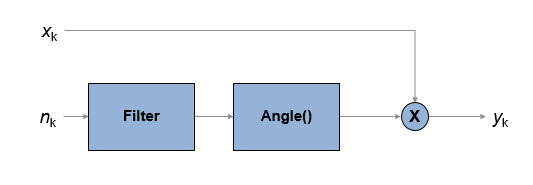# comm.PhaseNoise

Apply phase noise to baseband signal

## Description

The `comm.PhaseNoise` System object™ adds phase noise to a complex signal. This object emulates impairments introduced by the local oscillator of a wireless communication transmitter or receiver. The object generates filtered phase noise according to the specified spectral mask and adds it to the input signal. For a description of the phase noise modeling, see Algorithms.

To add phase noise using a `comm.PhaseNoise` object:

1. Create the `comm.PhaseNoise` object and set its properties.

2. Call the object with arguments, as if it were a function.

## Creation

### Syntax

``phznoise = comm.PhaseNoise``
``phznoise = comm.PhaseNoise(Name,Value)``
``phznoise = comm.PhaseNoise(level,offset,samplerate)``

### Description

````phznoise = comm.PhaseNoise` creates a phase noise System object with default property values.```

example

````phznoise = comm.PhaseNoise(Name,Value)` creates a phase noise object with the specified property `Name` set to the specified `Value`. You can specify additional name-value pair arguments in any order as (`Name1`,`Value1`,...,`NameN`,`ValueN`).```
````phznoise = comm.PhaseNoise(level,offset,samplerate)` creates a phase noise object with the phase noise level, frequency offset, and sample rate properties specified as value-only arguments. When specifying a value-only argument, you must specify all preceding value-only arguments.```

## Properties

expand all

Unless otherwise indicated, properties are nontunable, which means you cannot change their values after calling the object. Objects lock when you call them, and the `release` function unlocks them.

If a property is tunable, you can change its value at any time.

Phase noise level in decibels relative to carrier per hertz (dBc/Hz), specified as a vector of negative scalars. The `Level` and `FrequencyOffset` properties must have the same length.

Data Types: `double`

Frequency offset in Hz, specified as a vector of positive increasing values. The maximum frequency offset value must be less than FS/ 2, where FS represents the `SampleRate` property value.

The `Level` and `FrequencyOffset` properties must have the same length.

Data Types: `double`

Sample rate in Hz, specified as a positive scalar greater than two times the maximum value specified by the `FrequencyOffset` property.

Data Types: `double`

Source of the random stream, specified as `'Global stream'` or `'mt19937ar with seed'`.If `RandomStream` is set to `'mt19937ar with seed'`, the mt19937ar algorithm is used for normally distributed random number generation, in which case the reset method reinitializes the random number stream to the value of the `Seed` property.

Data Types: `char` | `string`

Initial seed for `RandomStream`, specified as a positive scalar less than 232.

#### Dependencies

To enable this property, set `RandomStream` to `'mt19937ar with seed'`.

Data Types: `double`

## Usage

### Syntax

``out = phznoise(in)``

### Description

example

````out = phznoise(in)` adds phase noise, specified by the `phznoise` System object, to the input signal. The result is returned in `out`.```

### Input Arguments

expand all

Input signal, specified as an NS-by-1 numeric vector or NS-by-M numeric matrix. NS is the number of samples and M is the number of channels.

Data Types: `double` | `single`
Complex Number Support: Yes

### Output Arguments

expand all

Output signal, returned as a complex-valued signal with the same data type and size as the input signal.

## Object Functions

To use an object function, specify the System object as the first input argument. For example, to release system resources of a System object named `obj`, use this syntax:

`release(obj)`

expand all

 `visualize` Visualize spectrum mask of phase noise
 `step` Run System object algorithm `release` Release resources and allow changes to System object property values and input characteristics `reset` Reset internal states of System object

## Examples

collapse all

Add a phase noise vector and frequency offset vector to a 16-QAM signal. Then plot the signal.

Create a phase noise System object.

`pnoise = comm.PhaseNoise('Level',-50,'FrequencyOffset',20);`

Generate modulated symbols.

```M = 16; % From 16-QAM data = randi([0 M-1],1000,1); modData = qammod(data,M);```

Use `pnoise` to apply phase noise. Plot the impaired data.

```y = pnoise(modData); scatterplot(y)```View the effects of phase noise on a 10 MHz sine wave by using a spectrum analyzer. Adjust the resolution bandwidth of the spectrum analyzer to see its impact on the visualized spectral noise.

Initialize variables for the simulation.

```fc = 1e6; % Carrier frequency in Hz fs = 4e6; % Sample rate in Hz. phNzLevel = [-85 -118 -125 -145]; % in dBc/Hz phNzFreqOff = [1e3 9.5e3 19.5e3 195e3]; % in Hz Nspf = 6e6; % Number of Samples per frame freqSpan = 400e3; % in Hz, for spectrum computation```

Create sine wave, phase noise, and spectrum analyzer objects.

```sinewave = dsp.SineWave( ... Amplitude=1, ... Frequency=fc, ... SampleRate=fs, ... SamplesPerFrame=Nspf, ... ComplexOutput=true); pnoise = comm.PhaseNoise( ... Level=phNzLevel, ... FrequencyOffset=phNzFreqOff, ... SampleRate=fs); sascopeRBW100 = spectrumAnalyzer( ... SampleRate=fs, ... Method="welch", ... FrequencySpan="Span and center frequency", ... CenterFrequency=fc, ... Span=freqSpan, ... RBWSource="Property", ... RBW=100, ... SpectrumType="Power density", ... SpectralAverages=10, ... SpectrumUnits="dBW", ... YLimits=[-150 10], ... Title="Resolution Bandwidth 100 Hz", ... ChannelNames={'signal','signal with phase noise'}, ... Position=[79 147 605 374]); sascopeRBW1k = spectrumAnalyzer( ... SampleRate=fs, ... Method="welch", ... FrequencySpan="Span and center frequency", ... CenterFrequency=fc, ... Span=freqSpan, ... RBWSource="Property", ... RBW=1000, ... SpectrumType="Power density", ... SpectralAverages=10, ... SpectrumUnits="dBW", ... YLimits=[-150 10], ... Title="Resolution Bandwidth 1 kHz", ... ChannelNames={'signal','signal with phase noise'}, ... Position=[685 146 605 376]);```

To analyze the spectrum and phase noise, the example includes two spectrum analyzer objects, with 100 Hz and 1 kHz resolution bandwidths, respectively. The spectrum analyzer objects use the default `Hann` windowing setting, the spectrum units are set to `dBW`, and the number of spectral averages is set to `10`.

```x = sinewave(); y = pnoise(x);```

When the resolution bandwidth is 100 Hz, the `dBW/Hz` view for the spectrum analyzer shows the tone at -20 dBW/Hz. The spectrum analyzer object corrects for the power spreading effect of the Hann windowing. Results show the visual average of the phase noise match the specified phase noise spectrum.

```sascopeRBW100(x,y) release(sascopeRBW100)```When the resolution bandwidth is 1 kHz, the `dBW/Hz` view for the spectrum analyzer shows the tone at -30 dBW/Hz. The tone energy of the sine wave is now spread across 1 kHz instead of 100 Hz, so the sine wave PSD level reduces by 10 dB. With the resolution bandwidth at 1 kHz, the visual average of the phase noise still achieves the phase noise defined by the phase noise object.

With the resolution bandwidth increased from 100 Hz to 1 kHz, the spectrum analyzer object still corrects for the power spreading effect of the Hann window, and it achieves better spectral averaging with the wider resolution bandwidth. For more information, see Why Use Windows?

```sascopeRBW1k(x,y) release(sascopeRBW1k)```Calculate the RMS phase noise in degrees between the pure and noisy sine waves. In the general case, the pure signal must be time aligned with the noisy signal to accurately determine the phase error. However, in this case, the periodicity of the sine wave makes this step unnecessary.

```ph_err = unwrap(angle(y) - angle(x)); rms_ph_nz_deg = rms(ph_err)*180/pi(); sprintf('The computed RMS phase noise is %3.2f degrees.', ... rms_ph_nz_deg)```
```ans = 'The computed RMS phase noise is 0.37 degrees.' ```

## Algorithms

expand all

The output signal, yk, is related to input sequence xk by yk=xkejφk, where φk is the phase noise. The phase noise is filtered Gaussian noise such that φk=f(nk), where nk is the noise sequence and f represents a filtering operation.To model the phase noise, define the power spectrum density (PSD) mask characteristic by specifying scalar or vector values for the frequency offset and phase noise level.

• For a scalar frequency offset and phase noise level specification, an IIR digital filter computes the spectrum mask. The spectrum mask has a 1 / f characteristic that passes through the specified point. For more information, see IIR Digital Filter.

• For a vector frequency offset and phase noise level specification, an FIR filter computes the spectrum mask. The spectrum mask is interpolated across log10(f). For more information, see FIR Filter.

 Kasdin, N. J., "Discrete Simulation of Colored Noise and Stochastic Processes and 1/(f^alpha); Power Law Noise Generation." The Proceedings of the IEEE. Vol. 83, No. 5, May, 1995, pp 802–827.